GeeksforGeeks App
Open AppBrowser
Continue

# Understanding TF-IDF (Term Frequency-Inverse Document Frequency)

TF-IDF stands for Term Frequency Inverse Document Frequency of records. It can be defined as the calculation of how relevant a word in a series or corpus is to a text. The meaning increases proportionally to the number of times in the text a word appears but is compensated by the word frequency in the corpus (data-set).

Terminologies:

• Term Frequency: In document d, the frequency represents the number of instances of a given word t. Therefore, we can see that it becomes more relevant when a word appears in the text, which is rational. Since the ordering of terms is not significant, we can use a vector to describe the text in the bag of term models. For each specific term in the paper, there is an entry with the value being the term frequency.

The weight of a term that occurs in a document is simply proportional to the term frequency.

`tf(t,d) = count of t in d / number of words in d`
• Document Frequency: This tests the meaning of the text, which is very similar to TF, in the whole corpus collection. The only difference is that in document d, TF is the frequency counter for a term t, while df is the number of occurrences in the document set N of the term t. In other words, the number of papers in which the word is present is DF.
`df(t) = occurrence of t in documents`
• Inverse Document Frequency: Mainly, it tests how relevant the word is. The key aim of the search is to locate the appropriate records that fit the demand. Since tf considers all terms equally significant, it is therefore not only possible to use the term frequencies to measure the weight of the term in the paper. First, find the document frequency of a term t by counting the number of documents containing the term:
```df(t) = N(t)
where
df(t) = Document frequency of a term t
N(t) = Number of documents containing the term t```

Term frequency is the number of instances of a term in a single document only; although the frequency of the document is the number of separate documents in which the term appears, it depends on the entire corpus. Now let’s look at the definition of the frequency of the inverse paper. The IDF of the word is the number of documents in the corpus separated by the frequency of the text.

`idf(t) = N/ df(t) = N/N(t)`

The more common word is supposed to be considered less significant, but the element (most definite integers) seems too harsh. We then take the logarithm (with base 2) of the inverse frequency of the paper. So the if of the term t becomes:

`idf(t) = log(N/ df(t))`
• Computation: Tf-idf is one of the best metrics to determine how significant a term is to a text in a series or a corpus. tf-idf is a weighting system that assigns a weight to each word in a document based on its term frequency (tf) and the reciprocal document frequency (tf) (idf). The words with higher scores of weight are deemed to be more significant.

Usually, the tf-idf weight consists of two terms-

1. Normalized Term Frequency (tf)
2. Inverse Document Frequency (idf)
`tf-idf(t, d) = tf(t, d) * idf(t)`

In python tf-idf values can be computed using TfidfVectorizer() method in sklearn module.

Syntax:

sklearn.feature_extraction.text.TfidfVectorizer(input)

Parameters:

• input: It refers to parameter document passed, it can be a filename, file or content itself.

Attributes:

• vocabulary_: It returns a dictionary of terms as keys and values as feature indices.
• idf_: It returns the inverse document frequency vector of the document passed as a parameter.

Returns:

• fit_transform(): It returns an array of terms along with tf-idf values.
• get_feature_names(): It returns a list of feature names.

Step-by-step Approach:

• Import modules.

## Python3

 `# import required module``from` `sklearn.feature_extraction.text ``import` `TfidfVectorizer`

• Collect strings from documents and create a corpus having a collection of strings from the documents d0, d1, and d2.

## Python3

 `# assign documents``d0 ``=` `'Geeks for geeks'``d1 ``=` `'Geeks'``d2 ``=` `'r2j'` `# merge documents into a single corpus``string ``=` `[d0, d1, d2]`

• Get tf-idf values from fit_transform() method.

## Python3

 `# create object``tfidf ``=` `TfidfVectorizer()` `# get tf-df values``result ``=` `tfidf.fit_transform(string)`

• Display idf values of the words present in the corpus.

## Python3

 `# get idf values``print``(``'\nidf values:'``)``for` `ele1, ele2 ``in` `zip``(tfidf.get_feature_names(), tfidf.idf_):``    ``print``(ele1, ``':'``, ele2)`

Output: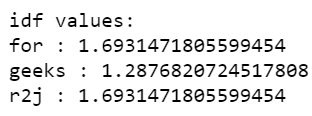• Display tf-idf values along with indexing.

## Python3

 `# get indexing``print``(``'\nWord indexes:'``)``print``(tfidf.vocabulary_)` `# display tf-idf values``print``(``'\ntf-idf value:'``)``print``(result)` `# in matrix form``print``(``'\ntf-idf values in matrix form:'``)``print``(result.toarray())`

Output: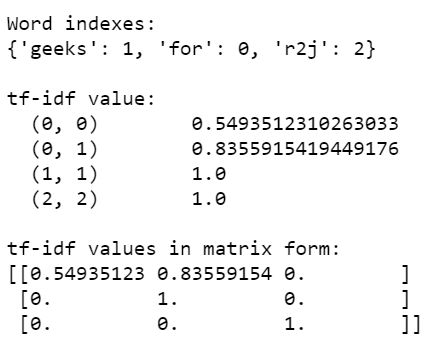The result variable consists of unique words as well as the tf-if values. It can be elaborated using the below image: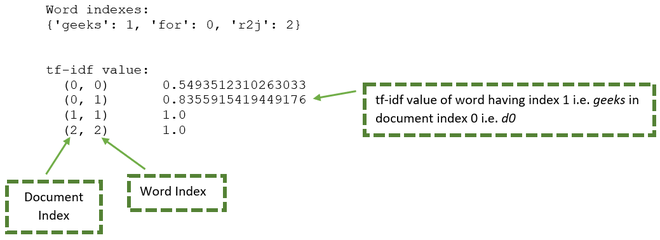From the above image the below table can be generated:

Below are some examples which depict how to compute tf-idf values of words from a corpus:

Example 1: Below is the complete program based on the above approach:

## Python3

 `# import required module``from` `sklearn.feature_extraction.text ``import` `TfidfVectorizer` `# assign documents``d0 ``=` `'Geeks for geeks'``d1 ``=` `'Geeks'``d2 ``=` `'r2j'` `# merge documents into a single corpus``string ``=` `[d0, d1, d2]` `# create object``tfidf ``=` `TfidfVectorizer()` `# get tf-df values``result ``=` `tfidf.fit_transform(string)` `# get idf values``print``(``'\nidf values:'``)``for` `ele1, ele2 ``in` `zip``(tfidf.get_feature_names(), tfidf.idf_):``    ``print``(ele1, ``':'``, ele2)` `# get indexing``print``(``'\nWord indexes:'``)``print``(tfidf.vocabulary_)` `# display tf-idf values``print``(``'\ntf-idf value:'``)``print``(result)` `# in matrix form``print``(``'\ntf-idf values in matrix form:'``)``print``(result.toarray())`

Output: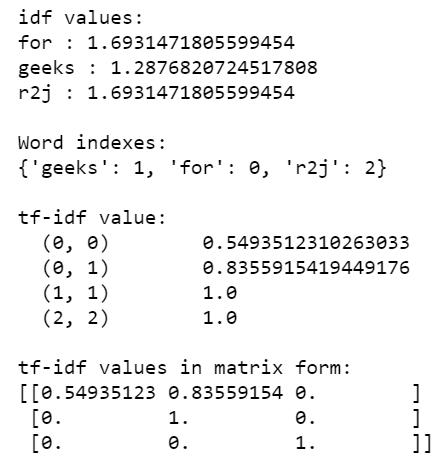Example 2: Here, tf-idf values are computed from a corpus having unique values.

## Python3

 `# import required module``from` `sklearn.feature_extraction.text ``import` `TfidfVectorizer` `# assign documents``d0 ``=` `'geek1'``d1 ``=` `'geek2'``d2 ``=` `'geek3'``d3 ``=` `'geek4'` `# merge documents into a single corpus``string ``=` `[d0, d1, d2, d3]` `# create object``tfidf ``=` `TfidfVectorizer()` `# get tf-df values``result ``=` `tfidf.fit_transform(string)` `# get indexing``print``(``'\nWord indexes:'``)``print``(tfidf.vocabulary_)` `# display tf-idf values``print``(``'\ntf-idf values:'``)``print``(result)`

Output: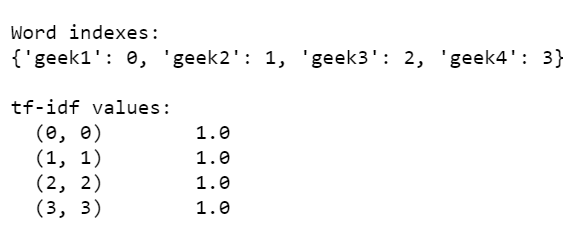Example 3: In this program, tf-idf values are computed from a corpus having similar documents.

## Python3

 `# import required module``from` `sklearn.feature_extraction.text ``import` `TfidfVectorizer` `# assign documents``d0 ``=` `'Geeks for geeks!'``d1 ``=` `'Geeks for geeks!'`  `# merge documents into a single corpus``string ``=` `[d0, d1]` `# create object``tfidf ``=` `TfidfVectorizer()` `# get tf-df values``result ``=` `tfidf.fit_transform(string)` `# get indexing``print``(``'\nWord indexes:'``)``print``(tfidf.vocabulary_)` `# display tf-idf values``print``(``'\ntf-idf values:'``)``print``(result)`

Output: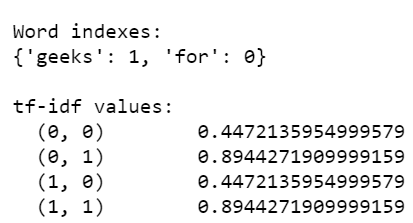Example 4: Below is the program in which we try to calculate tf-idf value of a single word geeks is repeated multiple times in multiple documents.

## Python3

 `# import required module``from` `sklearn.feature_extraction.text ``import` `TfidfVectorizer` `# assign corpus``string ``=` `[``'Geeks geeks'``]``*``5` `# create object``tfidf ``=` `TfidfVectorizer()` `# get tf-df values``result ``=` `tfidf.fit_transform(string)` `# get indexing``print``(``'\nWord indexes:'``)``print``(tfidf.vocabulary_)` `# display tf-idf values``print``(``'\ntf-idf values:'``)``print``(result)`

Output: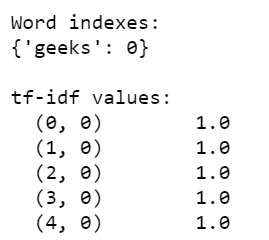My Personal Notes arrow_drop_up